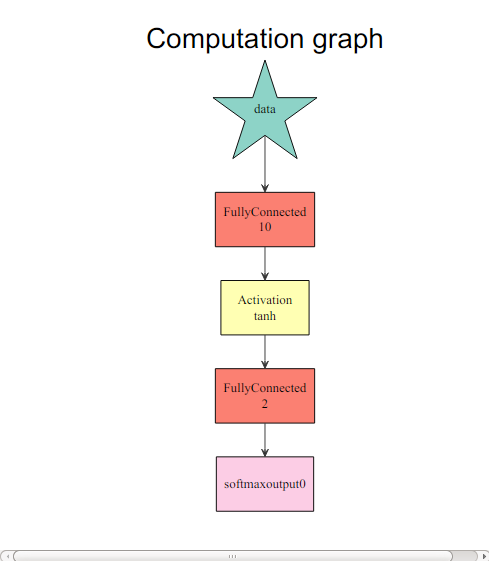# Develop a Neural Network with MXNet in Five Minutes

This tutorial is designed for new users of the `mxnet` package for R. It shows how to construct a neural network to do regression in 5 minutes. It shows how to perform classification and regression tasks, respectively. The data we use is in the `mlbench` package. Instructions to install R and MXNet's R package in different environments can be found here.

## Classification

``````    ## Loading required package: mlbench
``````
``````    if (!require(mlbench)) {
install.packages('mlbench')
}
``````
``````    ## Loading required package: mxnet
``````
``````    require(mxnet)
``````
``````    ## Loading required datasets
``````
``````    data(Sonar, package="mlbench")

Sonar[,61] = as.numeric(Sonar[,61])-1
train.ind = c(1:50, 100:150)
train.x = data.matrix(Sonar[train.ind, 1:60])
train.y = Sonar[train.ind, 61]
test.x = data.matrix(Sonar[-train.ind, 1:60])
test.y = Sonar[-train.ind, 61]
``````

We are going to use a multi-layer perceptron as our classifier. In `mxnet`, we have a function called `mx.mlp` for building a general multi-layer neural network to do classification or regression.

`mx.mlp` requires the following parameters:

• Training data and label
• Number of hidden nodes in each hidden layer
• Number of nodes in the output layer
• Type of the activation
• Type of the output loss
• The device to train (GPU or CPU)
• Other parameters for `mx.model.FeedForward.create`

The following code shows an example usage of `mx.mlp`:

``````    mx.set.seed(0)
model <- mx.mlp(train.x, train.y, hidden_node=10, out_node=2, out_activation="softmax",
num.round=20, array.batch.size=15, learning.rate=0.07, momentum=0.9,
eval.metric=mx.metric.accuracy)
``````
``````    ## Auto detect layout of input matrix, use rowmajor..
## Start training with 1 devices
##  Train-accuracy=0.488888888888889
##  Train-accuracy=0.514285714285714
##  Train-accuracy=0.514285714285714
##  Train-accuracy=0.514285714285714
##  Train-accuracy=0.514285714285714
##  Train-accuracy=0.523809523809524
##  Train-accuracy=0.619047619047619
##  Train-accuracy=0.695238095238095
##  Train-accuracy=0.695238095238095
##  Train-accuracy=0.761904761904762
##  Train-accuracy=0.828571428571429
##  Train-accuracy=0.771428571428571
##  Train-accuracy=0.742857142857143
##  Train-accuracy=0.733333333333333
##  Train-accuracy=0.771428571428571
##  Train-accuracy=0.847619047619048
##  Train-accuracy=0.857142857142857
##  Train-accuracy=0.838095238095238
##  Train-accuracy=0.838095238095238
##  Train-accuracy=0.838095238095238
``````

Note that `mx.set.seed` controls the random process in `mxnet`. You can see the accuracy in each round during training. It's also easy to make predictions and evaluate.

To get an idea of what is happening, view the computation graph from R:

``````    graph.viz(model\$symbol)
````````````    preds = predict(model, test.x)
``````
``````    ## Auto detect layout of input matrix, use rowmajor.
``````
``````    pred.label = max.col(t(preds))-1
table(pred.label, test.y)
``````
``````    ##           test.y
## pred.label  0  1
##          0 24 14
##          1 36 33
``````

Note for that for multi-class predictions, mxnet outputs `nclass` x `nexamples`, with each row corresponding to the probability of the class.

## Regression

Again, let us preprocess the data:

``````    data(BostonHousing, package="mlbench")

train.ind = seq(1, 506, 3)
train.x = data.matrix(BostonHousing[train.ind, -14])
train.y = BostonHousing[train.ind, 14]
test.x = data.matrix(BostonHousing[-train.ind, -14])
test.y = BostonHousing[-train.ind, 14]
``````

Although we can use `mx.mlp` again to do regression by changing the `out_activation`, this time we are going to introduce a flexible way to configure neural networks in `mxnet`. Configuration is done by the "Symbol" system in `mxnet`. The Symbol system takes care of the links among nodes, activation, dropout ratio, etc. Configure a multi-layer neural network as follows:

``````    # Define the input data
data <- mx.symbol.Variable("data")
# A fully connected hidden layer
# data: input source
# num_hidden: number of neurons in this hidden layer
fc1 <- mx.symbol.FullyConnected(data, num_hidden=1)

# Use linear regression for the output layer
lro <- mx.symbol.LinearRegressionOutput(fc1)
``````

What matters for a regression task is mainly the last function. It enables the new network to optimize for squared loss. Now let's train on this simple data set. In this configuration, we dropped the hidden layer so that the input layer is directly connected to the output layer.

Next, make prediction with this structure and other parameters with `mx.model.FeedForward.create`:

``````    mx.set.seed(0)
model <- mx.model.FeedForward.create(lro, X=train.x, y=train.y,
ctx=mx.cpu(),     num.round=50, array.batch.size=20,
learning.rate=2e-6, momentum=0.9,  eval.metric=mx.metric.rmse)
``````
``````    ## Auto detect layout of input matrix, use rowmajor.
## Start training with 1 devices
##  Train-rmse=16.063282524034
##  Train-rmse=12.2792375712573
##  Train-rmse=11.1984634005885
##  Train-rmse=10.2645236892904
##  Train-rmse=9.49711005504284
##  Train-rmse=9.07733734175182
##  Train-rmse=9.07884450847991
##  Train-rmse=9.10463850277417
##  Train-rmse=9.03977049028532
##  Train-rmse=8.96870685004475
##  Train-rmse=8.93113287361574
##  Train-rmse=8.89937257821847
##  Train-rmse=8.87182096922953
##  Train-rmse=8.84476075083586
##  Train-rmse=8.81464673014974
##  Train-rmse=8.78672567900196
##  Train-rmse=8.76265872846474
##  Train-rmse=8.73946101419974
##  Train-rmse=8.71651926303267
##  Train-rmse=8.69457600919277
##  Train-rmse=8.67354928674563
##  Train-rmse=8.65328755392436
##  Train-rmse=8.63378039680078
##  Train-rmse=8.61488162586984
##  Train-rmse=8.5965105183022
##  Train-rmse=8.57868133563275
##  Train-rmse=8.56135851937663
##  Train-rmse=8.5444819772098
##  Train-rmse=8.52802114610432
##  Train-rmse=8.5119504512622
##  Train-rmse=8.49624261719241
##  Train-rmse=8.48087453238701
##  Train-rmse=8.46582689119887
##  Train-rmse=8.45107881002491
##  Train-rmse=8.43661331401712
##  Train-rmse=8.42241575909639
##  Train-rmse=8.40847217331365
##  Train-rmse=8.39476931796395
##  Train-rmse=8.38129658373974
##  Train-rmse=8.36804269059018
##  Train-rmse=8.35499817678397
##  Train-rmse=8.34215505742154
##  Train-rmse=8.32950441908131
##  Train-rmse=8.31703985777311
##  Train-rmse=8.30475363906755
##  Train-rmse=8.29264031506106
##  Train-rmse=8.28069372820073
##  Train-rmse=8.26890902770415
##  Train-rmse=8.25728089053853
##  Train-rmse=8.24580511500735
``````

It's also easy to make a prediction and evaluate it:

``````    preds = predict(model, test.x)
``````
``````    ## Auto detect layout of input matrix, use rowmajor..
``````
``````    sqrt(mean((preds-test.y)^2))
``````
``````    ##  7.800502
``````

Currently, we have four predefined metrics: "accuracy", "rmse", "mae", and "rmsle". MXNet provides the interface for defining your own metrics:

``````    demo.metric.mae <- mx.metric.custom("mae", function(label, pred) {
pred <- mx.nd.reshape(pred, shape = 0)
res <- mx.nd.mean(mx.nd.abs(label-pred))
return(res)
})
``````

This is an example of the mean absolute error metric. Simply plug it into the training function:

``````    mx.set.seed(0)
model <- mx.model.FeedForward.create(lro, X=train.x, y=train.y,
ctx=mx.cpu(),    num.round=50, array.batch.size=20,
learning.rate=2e-6, momentum=0.9, eval.metric=demo.metric.mae)
``````
``````    ## Auto detect layout of input matrix, use rowmajor.
## Start training with 1 devices
##  Train-mae=14.953625731998
##  Train-mae=11.4802955521478
##  Train-mae=8.50700579749213
##  Train-mae=7.30591265360514
##  Train-mae=7.38049803839789
##  Train-mae=7.36036252975464
##  Train-mae=7.06519222259521
##  Train-mae=6.9962231847975
##  Train-mae=6.96296903822157
##  Train-mae=6.9046172036065
##  Train-mae=6.87867620256212
##  Train-mae=6.85872554779053
##  Train-mae=6.81936407089233
##  Train-mae=6.79135354359945
##  Train-mae=6.77438741260105
##  Train-mae=6.75365140702989
##  Train-mae=6.73369296391805
##  Train-mae=6.71600982877943
##  Train-mae=6.69932826360067
##  Train-mae=6.6852519777086
##  Train-mae=6.67343420452542
##  Train-mae=6.66315894656711
##  Train-mae=6.65314838621351
##  Train-mae=6.64388704299927
##  Train-mae=6.63480265935262
##  Train-mae=6.62583245171441
##  Train-mae=6.61697626113892
##  Train-mae=6.60842116673787
##  Train-mae=6.60040124257406
##  Train-mae=6.59264140658908
##  Train-mae=6.58551020092434
##  Train-mae=6.57864215638902
##  Train-mae=6.57178926467896
##  Train-mae=6.56495311525133
##  Train-mae=6.55813185373942
##  Train-mae=6.5513252152337
##  Train-mae=6.54453214009603
##  Train-mae=6.53775374094645
##  Train-mae=6.53098879920112
##  Train-mae=6.52423816257053
##  Train-mae=6.51764053768582
##  Train-mae=6.51121346155802
##  Train-mae=6.5047902001275
##  Train-mae=6.49837123023139
##  Train-mae=6.49216641320123
##  Train-mae=6.48598252402412
##  Train-mae=6.4798010720147
##  Train-mae=6.47362396452162
##  Train-mae=6.46745183732775
##  Train-mae=6.46128723356459
``````

Congratulations! You've learned the basics for using MXNet in R. To learn how to use MXNet's advanced features, see the other tutorials.